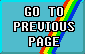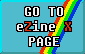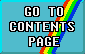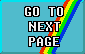# FIRST STEPS IN MACHINE CODE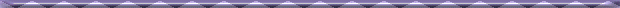## PART 3 - USING FLAGS#### An introduction to Z80 Machine Code by David Nowotnik.

One of the essential features of all computer programming languages is the ability to test a condition, and take different courses of action according to the result of that test (IF ... THEN ... in BASIC). Machine code is no exception, and in this article I shall be examining how the Z8O processor makes decisions. There will also be a little on simple arithmetic, and one of the Z80's powerful load instructions, which enables large chunks of memory to be moved with a few bytes of code.#### THE FLAGS

In part 1 of this series, I described how a register in the Z80 CPU, or a byte of memory, is made up. If you recall, there are eight bits within a register or byte, each bit has two states, 0 or 1. It is the combination of values of bits within a byte that makes up the value held by the register or byte.

The F register in the CPU is a special purpose register in which the individual bit values are far more important than the total byte value. 'F' stands for 'flag'; it is the bits of the F register which 'flag' messages to the processor, and to the machine code program. Following almost every single instruction carried out by the Z8O processor, the flag register is automatically modified according to the nature and result of the operation performed. In effect, the CPU tests the result of the operation, and the results of the test are recorded as yes/no answers (1 or 0) by certain bits (flags) within the F register. Not all the flags are of value to the machine code programmer; those that can be used are described below:

 The Carry (C) Flag This tests whether an addition or subtraction operation remained within the limits of a byte value (0 - 255), or if carry or borrow occurred. Other applications will be demonstrated later in the series. The Parity/Overflow (P/V) Flag Tests the parity of the result of an operation. This involves counting the 1's in the bits which form the result byte. If the parity sum is odd, then the P/V flag is set to 0. The flag is also used to demonstrate a 'carry' in two's complement arithmetic (also dealt with later on). The Zero (Z) Flag Set to 1 if the result of an operation is zero, otherwise this flag is set to 0. The Sign (S) Flag This takes the valued of the highest bit (bit 7) of a register following an operation on that register. Don't worry if most of what I have described isn't too clear yet, it should become clear later on in the series.

A programmer can set (flag value = 1) or reset (flag value = 0) any of these flags (either directly, with a specific machine code instruction, or indirectly with a 'dummy' operation). More often, the programmer will want to test whether a flag has a value of 1 or 0, and respond accordingly. That response usually means moving (or not moving) to another part of the program. In BASIC, this could be GOTO, or GOSUB (or RETurn from subroutine) if the condition is, or is not, met. The same choices are available in machine code. These are shown in the arithmetic and block move table.

##### VIEW ARITHMETIC AND BLOCK MOVE TABLES

There are two machine code instructions which are equivalent to BASIC's GOTO. These are JP and JR. JP stands for jump absolute, and JR for jump relative. JP instructions are usually three bytes long; the first is the opcode, the second and third contain the address in memory to which the program will jump. The address is calculated as the value in the second byte plus 256 times the value in the third byte. JR instructions are two bytes long; the first is the opcode, and the second is the distance (and direction) of the relative jump. The jump is calculated in exactly the same way as DJNZ, described last time. That is, the jump can be anywhere from -128 to +127 from the address of the opcode immediately following the JR instruction.

JR has the advantages that it uses two instead of three bytes, and the jump is independent of the position of the machine code in memory, so should be used in preference to JP. If the jump is greater than the limits set on JR, then JP is easier to use (it is not impossible to use JR for large jumps, but it could be tricky to program).

The equivalent of GOSUB in assembly language is CALL. It works in just the same way. The argument of CALL is an address in memory, so the instruction is three bytes long (the first for the opcode, the second and third the address in memory). Unfortunately, the Z80 processor does not allow relative CALLs. The subroutine is terminated with the RET instruction, which, like the RETurn command in BASIC, returns the operation of the program to the instruction immediately following the CALL instruction which initiated the subroutine.

JP, JR, CALL, and RET all have unconditional (i.e. not dependent on a test of any flag) as well as conditional options. Most of these are shown in the testing flags table.

##### VIEW TESTING FLAGS TABLE#### COMPARISONS

A simple example of using flags is in conjunction with the assembly language instruction CP (compare). This allows the contents of the A register to be compared with the contents of any other register, or the contents of a byte in memory (indexed by HL). In effect, with CP, the CPU carries out a subtraction between the A register contents, and the register or byte with which it is being compared. The result is not stored anywhere, but the result effects the values of the flags. For example, here is a typical assembly language sequence involving CP:

CP E
JR NZ, next

Having compared the value in the A register with that in the E register, the CPU sets or resets the zero (Z) flag according to the result. If A = E, then the Z flag = 1, and if A does not equal E, then the flag is reset to 0. The instruction 'JR NZ, next' tests the value of the Z flag. If the flag is not zero (NZ), then a relative jump to the position in the program labelled 'next' will occur; if the flag is set, then the program continues with the instruction immediately following 'JR NZ, next'. Other flags are set or reset according to the relative values of the two registers being compared. It is possible to test whether one is greater or less than the other. Rather than tell you all of these, I have given you a program which will show the effect of certain comparisons. This is the first example given later on in the article. By setting different values in the registers, you should be able to determine for yourself the flag settings for 'less than' or 'greater than' using this program. Why not try it!Like it or not, computer programs almost invariably involve some arithmetic, and machine code is no exception. The facilities for arithmetic on the Z80 processor are quite simple; addition, subtraction, and instructions (which I'll cover in a later issue) to effectively multiply and divide by two. All other mathematical operations are made up from these basic operations. Last time, I described the simple cases of adding or subtracting one (INC and DEC) from a register, or register pair. There are a number of machine code instructions which allow more complex addition and subtraction. For these, the choice of register is more limited than with INC and DEC. A single register addition or subtraction must always involve the 'A' register. Addition takes the form of adding the contents of the A register to another register or byte in memory (addressed by the HL pair), or a number (operand) in the program, and placing the result in the A register. Similarly, subtraction involves taking the value of a register, memory byte, or operand from the value in 'A' and placing the result back in the A register.

Two byte arithmetic is more restricted. Addition involves taking the contents of the HL register pair, adding the contents of another register, and placing the result in HL. Subtraction involves subtracting a register pair value from the value in HL, and placing the result in HL. In single byte arithmetic, numbers are restricted to the range 0 to 255, whilst the range for two byte arithmetic is 0 to 65535.

A further complication is the carry flag. It is possible to add two numbers, then add the value of carry (0 or 1) to the result just before storing the final value. And it is possible to subtract the complement (1 minus the value) of the carry flag from the result of subtracting two values, before storing the result. This gives two forms of add (ADD and ADC) and two forms of subtract (SUB and SBC); without and with the carry flag. Confused? I'll try and explain.

You'll see all the opcodes for the add and subtract in Table 2. Note that for two byte subtraction, only SBC is available, so be sure to set the carry flag when using it for simple arithmetic. Figure 2 in the examples section, later on, provides a BASIC program which should help to clarify any doubts about the different add and subtract instructions.The final piece of theory for this issue is the Z80's powerful block load instruction. It allows the values in a block of bytes (of any size) in memory to be moved to another location. It works like this:

The starting address of the source block is loaded into the HL register pair, the starting address of the destination block is moved into DE, and the number of bytes to be moved goes into BC. Then one instruction LDIR performs the move. LDIR stands for LoaD Increment Repeat. The value in BC acts as a counter. The value in the byte addressed by HL is transferred to the byte addressed by DE; HL and DE are incremented, while BC is decremented, then the instruction repeats itself automatically until BC gets down to zero. There is a non-repeat alternative, LDI, where the byte value transfer takes place, and registers HL and DE are incremented, BC decremented, but the instruction is not repeated automatically. Beginners to machine code would normally use LDIR, and this is shown in the examples.

The Z80 offers an almost identical instruction called LDDR. To use this, the END addresses of the source and destination blocks are loaded into HL and DE, respectively, while BC still holds the number of bytes to be transferred. Each cycle of the instruction involves transfer from (HL) to (DE), and all three register pairs decremented. LDD is the non-automatic repeat version of LDDR. It doesn't really matter whether you use LDIR or LDDR unless there is some overlap between the source and destination blocks of memory. Then, the choice is critical if bytes are not going to be corrupted. I'll leave you to sort out which should be used, depending on whether the overlap is at the beginning or end of the source block! The block move opcodes are shown in the arithmetic and block move table.#### EXAMPLES

Example 1 is the flag demonstration program. Example 2 demonstrates add and subtract, and should help explain any questions you might have on all the arithmetic instructions mentioned earlier. Figure 2 contains the details of this.

The final example carries out similar block move operations; copying the display file to a safe area of RAM, then returning it 'instantly'. Next time we'll be looking at a stack which has little to do with chimneys, and some bit operations!

Type in the listing shown in the DEMONSTRATION OF FLAGS PROGRAM. Once you have typed in the listing, SAVE the program, then RUN it. You'll get an INPUT request on the screen. This is the first part of the program, and you're expected to enter the machine code routine as a series of decimal numbers. Refer to the assembly language listing, below, and enter the numbers on the left hand side, in the order shown. Once you have entered all 22 numbers, enter -1, which informs the program that you have come to the end, If you made a mistake on entry then a checksum should detect it, and stop the program going any further. Once you enter the main routine, on screen instructions and display should be clear enough to make further description unnecessary.

There are three new machine code commands in the routine outlined below. They'll be covered in the next two parts of this series.

##### VIEW ASSEMBLY LANGUAGE LISTINGWhen this program is RUN, there is on the screen a diagrammatic representation of two pairs of bytes in memory (addressed by HL and DE), the 'A' register and the carry flag. The program asks you to insert two values (0 - 65535) into the pairs of bytes. The value is automatically split into low and high byte values, and placed in the appropriate box. You then select 1 or 0 for the carry flag, and the arithmetic operation (ADD, ADC, SUB, or SBC). A slow motion display then shows you what happens when you conduct two byte arithmetic using one byte instructions. The assembly language instruction appropriate to the operation being carried out will be shown at the bottom left of the screen. You should be able to see the effect on the carry flag and result of using the four different operations.